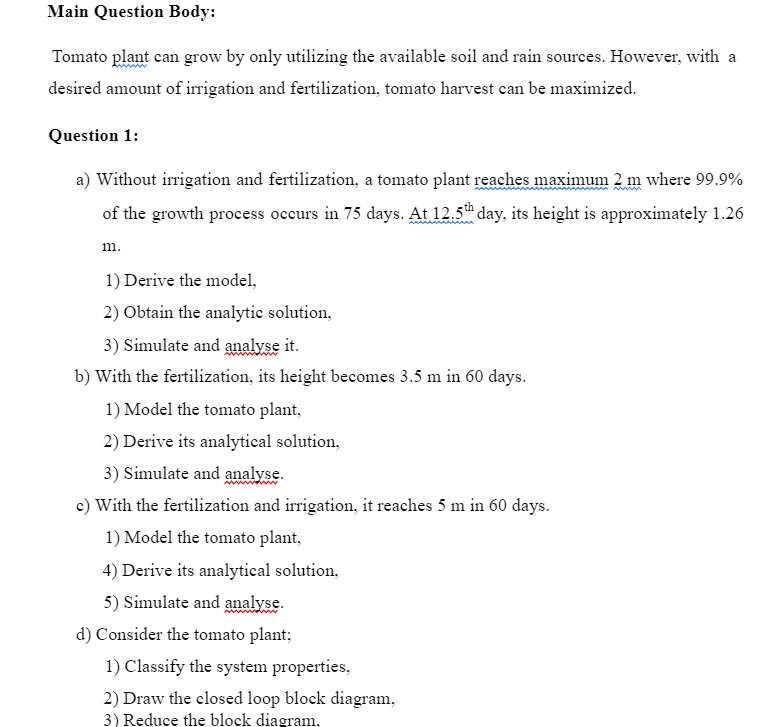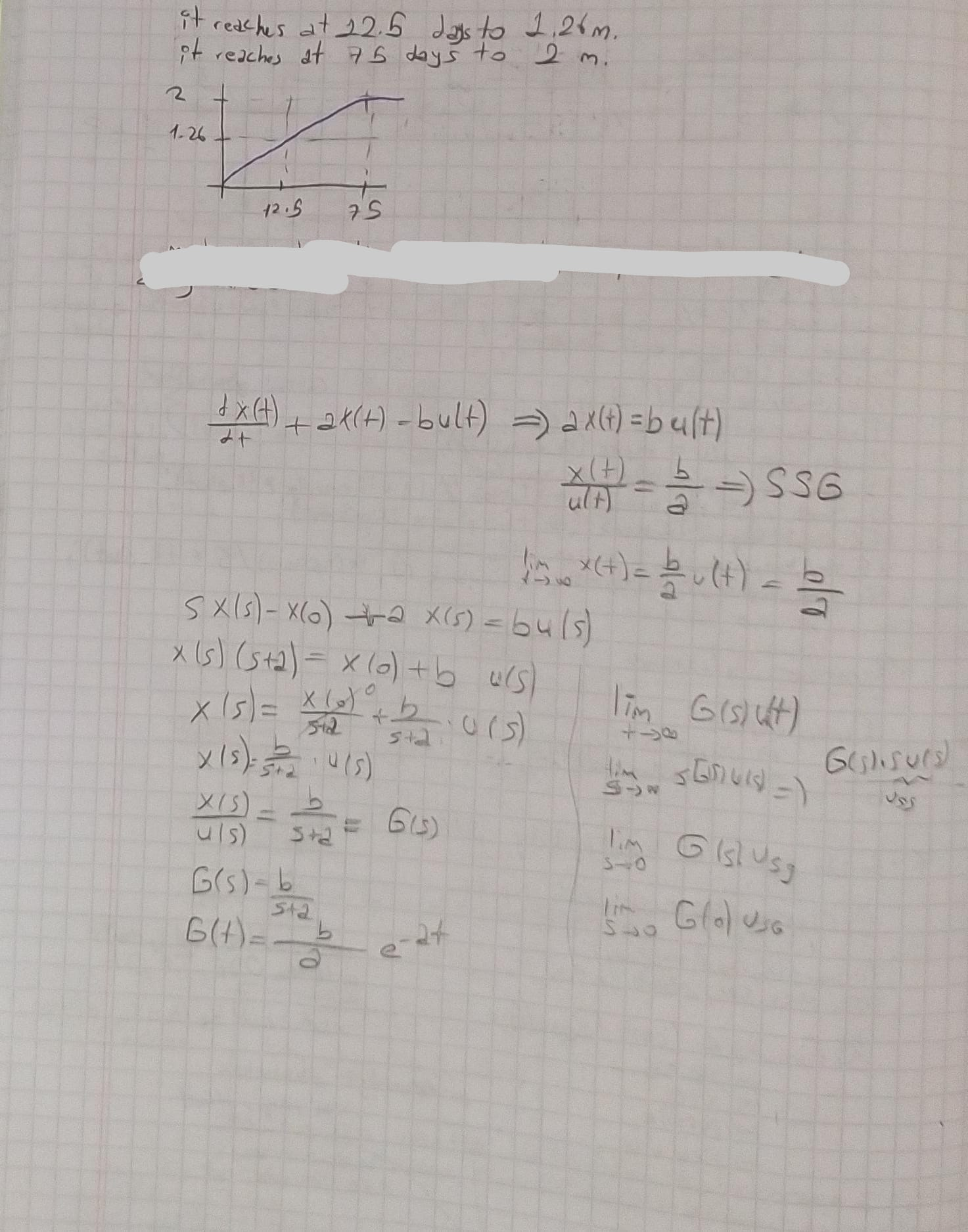# (Solved): use this equations(You can derive equations from these equations) and Help me about answer my Contro ...use this equations(You can derive equations from these equations) and Help me about answer my Control Theory Homework questions.

Main Question Body: Tomato plant can grow by only utilizing the available soil and rain sources. However, with a desired amount of irrigation and fertilization, tomato harvest can be maximized. Question 1: a) Without irrigation and fertilization, a tomato plant reaches maximum where of the growth process occurs in 75 days. At day, its height is approximately 1.26 . 1) Derive the model, 2) Obtain the analytic solution, 3) Simulate and analyse it. b) With the fertilization, its height becomes in 60 days. 1) Model the tomato plant, 2) Derive its analytical solution, 3) Simulate and analyse. c) With the fertilization and irrigation, it reaches in 60 days. 1) Model the tomato plant, 4) Derive its analytical solution, 5) Simulate and analyse. d) Consider the tomato plant; 1) Classify the system properties, 2) Draw the closed loop block diagram, 3) Reduce the block diagram. it reaches at 12.5 dass to . it reaches at 75 days to .

We have an Answer from Expert